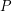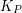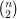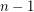# Partition of Complete Geometric Graph into Plane Trees

 Importance: Medium ✭✭
 Author(s):
 Subject: Geometry
 Keywords: complete geometric graph, edge colouring
 Recomm. for undergrads: no
 Posted by: David Wood on: October 19th, 2009
Conjecture   Every complete geometric graph with an even number of vertices has a partition of its edge set into plane (i.e. non-crossing) spanning trees.

For a setofpoints in the plane with no three collinear, the complete geometric graphhas vertex setand edge set consisting of thestraight line-segments between each pair of points in.

Since each subtree ofhas at mostedges, every partition ofinto subtrees has at leastparts. The conjecture asks for such a partition into exactlysubtrees, such that in addition, no two edges in each subtree cross.

It is folklore that the conjecture is true ifis in convex partition. In fact, the edge set of the complete convex graph can be partitioned into plane Hamiltonian paths. Bose et al. [BHRW] characterised all possible partitions of the complete convex graph into plane spanning trees. Bose et al. [BHRW] also proved that every complete geometric graph onvertices can be partitioned into at mostplane subtrees.

I heard about this conjecture from Ferran Hurtado in 2003, but the problem is much older than that.

## Bibliography

[BHRW] Prosenjit Bose, Ferran Hurtado, Eduardo Rivera-Campo, David R. Wood. Partitions of complete geometric graphs into plane trees, Computational Geometry: Theory & Applications 34(2):116-125, 2006. MathSciNet

* indicates original appearance(s) of problem.